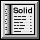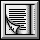Unit Cells

 NaCl Unit Cell The radii of the ions is: rNa+= 0.95 Ĺ and rCl-= 1.81 Ĺ. Since the sodium ion is 52.5% the size of the chloride ion, we can predict that the chloride ions will occupy the lattice points that define the face centered cubic unit cell, with the sodium ions occupying the octahedral holes. Notice that the octahedral holes are along the edges (for a net of three cations) and in the center of the cube (one more cation). Thus we have four anions (FCC) and four cations, which corresponds with the stoichiometry of the salt (1:1). Reset Crystal

 CsCl Unit Cell With a radius of 1.60 Ĺ, the cesium ion is 88.4% the size of the chloride ion. Based on this we can predict that the chloride ions will occupy the lattice points that define a simple cubic unit cell, while the cesium ion occupies the cubic hole at the center of the SC unit cell. Reset Crystal

 Li2Se Unit Cell The radii of the ions is: rLi+= 0.60 Ĺ and rSe2-= 1.98 Ĺ. Since the lithium ion is 30.3% of the selenide ion, we can predict that the selenide ions will occupy the lattice points that define the face centered cubic unit cell, with the lithium ions occupying the tetrahedral holes. Remember that the tetrahedral holes are found between each corner and the three adjacent faces. Therefore, there are eight tetrahedral holes inside each cube. Since the stoichiometry of the salt is 2:1, we must fill all of the tetrahedral holes (the FCC structure gives four selenides, thus we need eight lithiums). Reset Crystal

 ZnS Unit Cell The radius of the zinc ion is 35.0% that of the sulfide ion. Based on this, we can predict that the sulfide ions will occupy the latice points that define the face centered cubic unit cell, with the zinc ions occupying the tetrahedral holes. Since there are four sulfide ions (FCC), we need only four zinc ions to satisfy the stoichiometry. Therefore, only half of the eight tetrahedral holes inside of the unit cell will be filled. Notice that the filled holes form a tetrahedron themselves. Reset Crystal